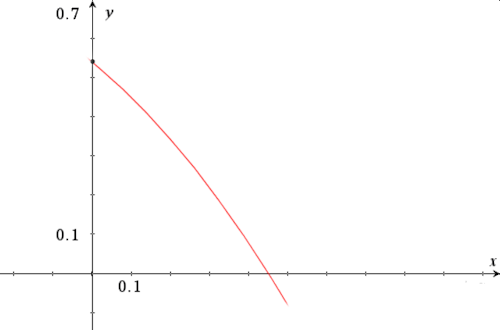# Exam-Style Questions.

## Problems adapted from questions set for previous Mathematics exams.

### 1.

IB Standard

The graph of $$f(x)=8-x^2$$ crosses the x-axis at the points A and B.

(a) Find the x-coordinate of A and of B.

(b) The region enclosed by the graph of $$f$$ and the x-axis is revolved 360o about the x-axis. Find the volume of the solid formed.

### 2.

IB Standard

The acceleration, $$a$$ ms-2 , of an object at time $$t$$ seconds is given by

$$a=\frac1t+4sin3t, (t\ge1)$$

The object is at rest when $$t=1$$.

Find the velocity of the object when $$t=7$$.

### 3.

IB Standard

Consider the graph of the function $$f(x)=x^2+2$$.

(a) Find the area between the graph of $$f$$ and the x-axis for $$2\le x \le 3$$.

(b) If the area described above is rotated 360o around the x-axis find the volume of the solid formed.

### 4.

A-Level

(a) Express the algebraic fraction

$$\frac{6x^2 - 47x + 49}{(5-x)(1-2x)}$$

in the form

$$A + \frac{B}{5-x} + \frac{C}{1-2x}$$

where $$A$$, $$B$$ and $$C$$ are integers.

(b) Hence show that the following integral equates to 3.03 correct to three significant figures.

$$\int^{0.25}_0 \frac{6x^2 - 47x + 49}{(5-x)(1-2x)} dx$$

### 5.

IB Standard

Make a sketch of a graph showing the velocity (in $$ms^{-1}$$) against time of a particle travelling for six seconds according to the equation:

$$v=e^{\sin t}-1$$

(a) Find the point at which the graph crosses the $$t$$ axis.

(b) How far does the particle travel during these first six seconds?

### 6.

IB Standard

Find the value of $$a$$ if $$\pi \lt a \lt 2\pi$$ and:

$$\int_\pi^a sin3x dx = -\frac13$$

### 7.

IB StandardThis graph represents the function $$f:x\to a \cos x, a\in \mathbf N$$

(a) Find the value of $$a$$.

(b) Find the area of the shaded region.

### 8.

IB Analysis and Approaches

Let $$f(x) = \frac{ln3x}{kx}$$ where $$x \gt 0$$ and $$k \in \mathbf Q^+$$.

(a) Find an expression for the first derivative $$f'(x)$$.

The graph of $$f$$ has exactly one maximum point at P.

(b) Find the x-coordinate of P.

The graph of $$f$$ has exactly one point of inflection at Q.

(c) Find the x-coordinate of Q.

(d) The region enclosed by the graph of $$f$$, the x-axis, and the vertical lines through P and Q has an area of one square unit, find the value of $$k$$.

### 9.

IB Standard

The following diagram shows the graph of $$f(x) = \cos(e^x) \; \text{for} \; 0 \le x \le 0.5$$.(a) Find the x-intercept of the graph of $$f(x)$$.

The region enclosed by the graph of $$f(x)$$, the y-axis and the x-axis is rotated 360° about the x-axis.

(b) Find the volume of the solid formed.

The exam-style questions appearing on this site are based on those set in previous examinations (or sample assessment papers for future examinations) by the major examination boards. The wording, diagrams and figures used in these questions have been changed from the originals so that students can have fresh, relevant problem solving practice even if they have previously worked through the related exam paper.

The solutions to the questions on this website are only available to those who have a Transum Subscription.

Exam-Style Questions Main Page

Search for exam-style questions containing a particular word or phrase:

To search the entire Transum website use the search box in the grey area below.